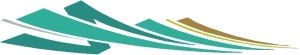### Electricity 101

written by Sterling

You'll see me toss around three major electrical concepts here: voltage, current, and power. They're all different, but related.

Voltage (measured in volts, imagine that) is the electrical equivalent of pressure. You know how a tire has a bunch of air pressure in it? That's like the voltage in a battery.

Current (measured in amps) is a measure of electrical flow. Imagine the air hose (that fills the tire); you can feel and hear the flow of air hissing out of the hose. That's like the current coming through a wire, charging the battery.

In order to charge a battery, you need more voltage in the wire than the battery has in it, the same way as a tire needs an air hose with higher pressure than it has - or air (current) will flow the other direction, out of the tire (battery).

OK, moving right along . . . Power (measured in watts) is a bit more abstract, but it's basically a way to get a handle on how much work your electricity can actually do. Watts are calculated by multiplying volts times amps, so 50 amps at 12 volts can do the same work as 5 amps at 120 volts (50A x 12V = 5A x 120V = 600W). In our tire analogy, it's like comparing a skinny, high-pressure bicycle tire to a big, low-pressure innertube. They might look very different, but have the same amount of air inside.

Finally, one additional concept: amp-hours is, as its name implies, a measure of current over time. If you use 10 amps for 8 hours, you'll have consumed 80 amp-hours (80Ah). This is how RV batteries are measured, simply telling you how much current you can use for how long, at the standard RV voltage of 12V.